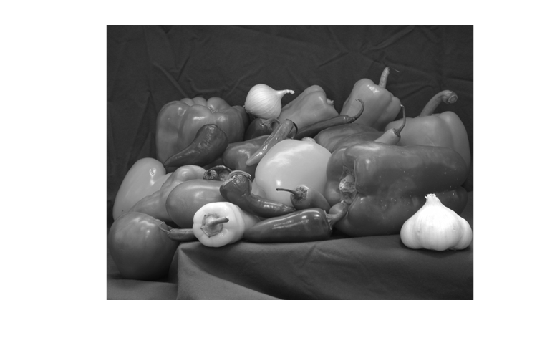# examples of imshow function in matlab

### All Contributions

Convert the RGB image to a grayscale image by using the `rgb2gray` function. and display gray Image

``````%Convert the RGB image to a grayscale image by using the rgb2gray function.
grayImage = rgb2gray(rgbImage);
%display grayImage
imshow(grayImage);``````

the resultRead a sample RGB image, `peppers.png`, into the MATLAB workspace.

``````rgbImage = imread("peppers.png");
%Display the RGB image using imshow
imshow(rgbImage);``````

the resultdisplay image

``imshow (I);``

imshow (I) displays the grayscale image I in a figure .

display image

``imshow (I);``

imshow (I) displays the grayscale image I in a figure .

total contributions (4)